Open in App
Not now

# Class 9 NCERT Solutions – Chapter 6 Lines And Angles – Exercise 6.1

• Difficulty Level : Medium
• Last Updated : 21 Jun, 2022

### Question 1. In the given figure, lines AB and CD intersect at O. If ∠AOC + ∠BOE = 70° and ∠BOD = 40°, find ∠BOE and reflex ∠COE?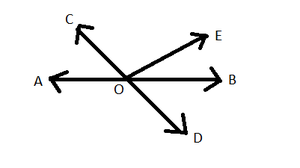Solution:

Given, AB and CD are straight lines.

∠AOC + ∠BOE = 70°  —-eq(i)

∠BOD = 40° —-eq(ii)

Since, AB is a straight line, the sum of all angles made on it is 180°

=> ∠AOC  + ∠COE + ∠BOE = 180° —eq(iii)

We can rearrange this equation as,

=> ∠AOC + ∠BOE + ∠COE = 180°

=> 70° + ∠COE = 180°  —from eq(i)

=> ∠COE = 180° – 70° = 110°

=> ∠COE = 110° —eq(iv)

Reflex ∠COE = 360° – ∠COE = 360° – 110° = 250°

Now, it is also given that CD is also a straight line, so the sum of all angles made on it is 180°

=> ∠COE + ∠BOE + ∠BOD = 180° —eq(v)

We can rearrange this equation as,

=> ∠COE + ∠BOD + ∠BOE = 180°

=> 110° + 40° + ∠BOE = 180°  —from eq(ii) and eq(iv)

=> 150° + ∠BOE = 180°

=> ∠BOE = 180° – 150° = 30°

=> ∠BOE = 30°

### Question 2. In the given figure, lines XY and MN intersect at O. If∠POY = 90° and a : b = 2 : 3, find c?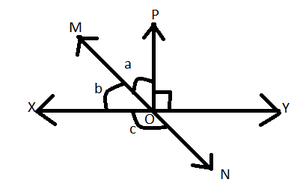Solution:

Given, XY and  MN are straight lines.

∠POY = 90° –eq(i)

a : b = 2 : 3 –eq(ii)

∠POM = a

∠XOM = b

∠XON = c

Taking XY as a straight line, so the sum of all angles made on it is 180°

=> ∠XOM + ∠POM + ∠POY = 180° —eq(iii)

=> b + a + 90° = 180°

=> 3x + 2x + 90° = 180° from eq(i) and eq(ii)

=> 5x + 90° = 180°

=> 5x = 180° – 90° = 90°

=> 5x = 90°

=> x = 18°

a : b = 2x : 3x = 2×18 : 3×18

a = 36°

b = 54°

Taking MN as a straight line so,the sum of all the angles made on it is 180°

=> ∠XOM  + ∠XON = 180°

=> 54° + ∠XON = 180°  from above finding value

=> ∠XON = 126° or c = 126°

### Question 3. In the given figure, ∠PQR = ∠PRQ, then prove that ∠PQS = ∠PRT?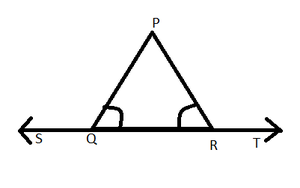Solution:

Given, ∠PQR = ∠PRQ

Taking ST is a straight line, so the sum of all angles made on it is 180°

=> ∠PQS + ∠PQR = 180° —-eq(i)

also,  ∠PRQ +  ∠PRT = 180° —eq(ii)

By equating both the equations because RHS of both the equation is equal So, LHS will also be equal.

=> ∠PQS +  ∠PQR  = ∠PRQ  + ∠PRT

=> ∠PQS + ∠PQR = ∠PQR + ∠PRT  –[ Given in question ∠PQR = ∠PRQ ]

=> ∠PQS = ∠PRT

### Question 4. In the given figure, if x + y = w + z, then prove that AOB is a line?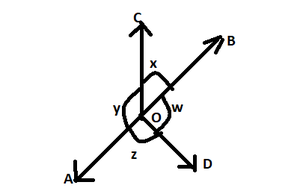Solution:

Given, x + y  = w + z  –eq(i)

We know that , sum of all angles made along  a point is 360°

So, Taking O as a point ∠AOC + ∠BOC + ∠BOD + ∠AOD = 360°

=> y + x + w + z = 360° from the given figure

=> (x + y) + (x + y) = 360°  from eq(i)

=> 2x + 2y = 360°

=> 2(x + y) = 360°

=> x + y=180°

From this statement it is proved that AOB is a straight line because the sum of angles made on the line is 180°. So, AOB is a straight line.

### Question 5. In the given figure, POQ is a line. Ray OR is perpendicular to line PQ. OS is another ray lying between rays OP and OR. Prove that∠ROS = (1/2) (∠QOS – ∠POS)?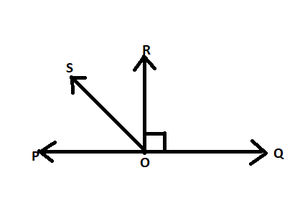Solution:

Given POQ is a straight line

So, the sum of all angles made on it is 180°

=> ∠POS + ∠ROS + ∠ROQ = 180°

=> ∠POS + ∠ROS + 90° = 180° [given ∠ROQ = 90°]

=> ∠POS + ∠ROS = 90°

=> ∠ROS = 90°  – ∠POS –eq(i)

Now, ∠ROS + ∠ROQ = ∠QOS   [from figure]

=> ∠ROS + 90° = ∠QOS

=> ∠ROS = ∠QOS – 90° –eq(ii)

Now Adding both the equations eq(i) + eq(ii)

=> ∠ROS + ∠ROS = 90° – ∠POS + ∠QOS – 90°

=> 2∠ROS =(∠QOS  – ∠POS)

=> ∠ROS = (1/2) (∠QOS – ∠POS)

Hence Verified!!!

### Question 6. It is given that ∠XYZ = 64° and XY is produced to point P. Draw a figure from the given information. If ray YQ bisects ∠ZYP, find ∠XYQ and reflex ∠QYP?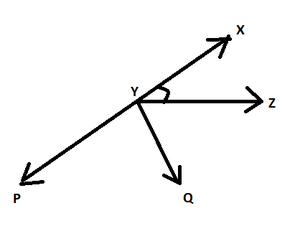Solution:

From the drawn figure, it is clearly shown that XYP is a straight line.

So, ∠XYZ + ∠ZYQ + ∠QYP = 180°

=> 64°+ ∠ZYQ + ∠QYP = 180°  [ given ∠XYZ = 64°]

=> 64° + 2∠QYP = 180°  [ YQ bisect ∠ZYP so, ∠QYP = ∠ZYQ]

=> 2∠QYP = 180° – 64° = 116°

=> ∠QYP = 58°

So, Reflex ∠QYP = 360° – 58° = 302°

Since ∠XYQ = ∠XYZ + ∠ZYQ

=> ∠XYQ = 64° + ∠QYP  [ given ∠XYZ = 64° and ∠ZYQ = ∠QYP]

=> ∠XYQ =64° + 58° = 122°

Thus, ∠XYQ = 122° and Reflex ∠QYP = 302°

My Personal Notes arrow_drop_up
Related Articles